# How to compare if multiple cells are equal in Excel?

To compare the values of two or more cells there are multiple formulas that can be used. For example, MATCH, If(A=B), EXACT, COUNTIF, etc. Here we will be learning the following two functions to find the exact match or where the formula will compare the strings without considering the case of strings.

• EXACT − To find the exact match.

• COUNTIF − To find similar values.

## Compare Multiple Cells for Equal Values

Step 1 − Following is the sample data that we have taken for comparing the strings/cell values in a datasheet.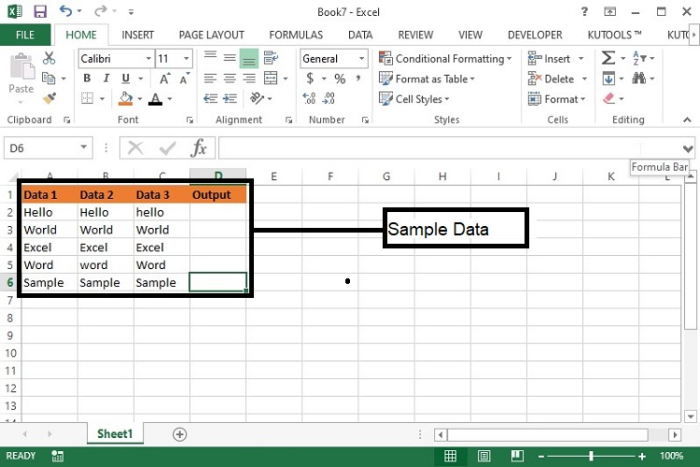Step 2 − Here, we have taken 3 columns with some similar data and some different data. In the 4th column the output will be displayed.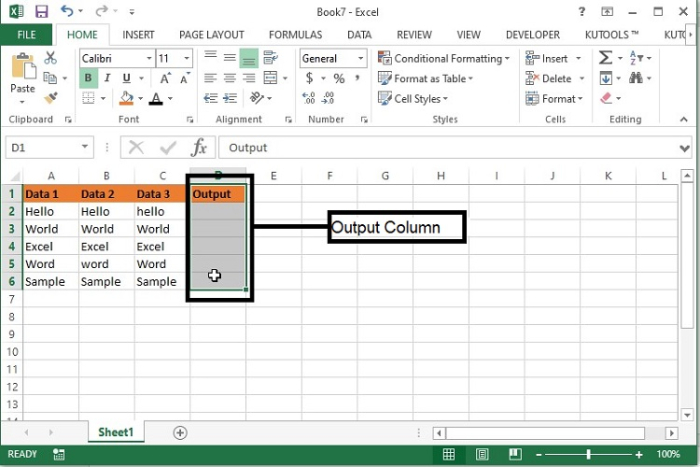Step 3 − Enter the following formula, in D2 cell and drag the same till the row which you want to copy the formula.

=AND(EXACT(A2−C2,A2))

Here, A2−C2 indicates the range of values and A2 indicates the starting value to compare.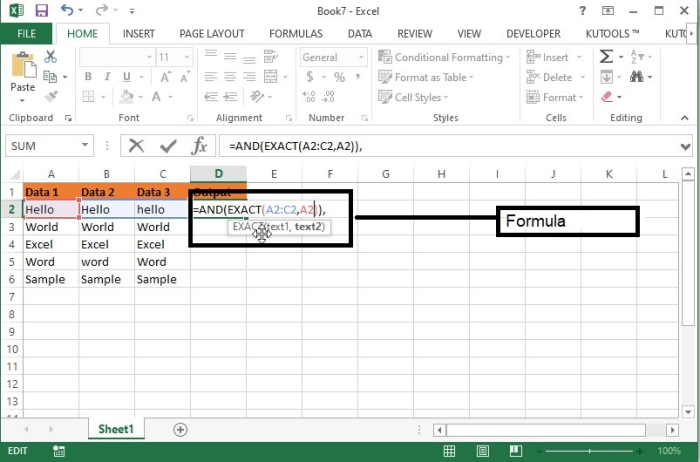Formula Syntax Description

Argument

Description

IF(logical_test, {value_if_true},{value_if_false}

• Logical_test specifies the condition basis which the data needs to be rendered.

• Value_if_true specifies the value that shall be returned if the condition satisfies.

• Value_if_false specifies the value that shall be returned if the condition does not satisfy.

Exact(Text1,Text2)

• Text1 this is the text with which other text to be compared.

• Text2 this is the text which needs to be compared with text1.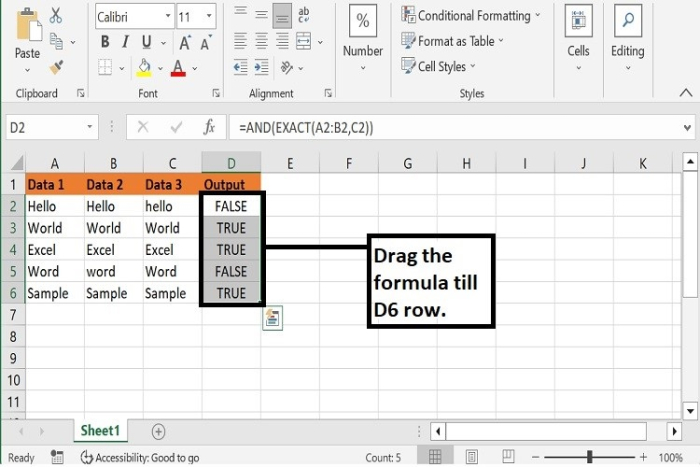## COUNTIF Formula

The EXACT formula return true only if the values matches exactly whereas, the COUNTIF function return TRUE if the values are similar irrespective of the case of the values. Let’s learn how to apply COUNTIF function.

Step 1 − Replace the above formula with the following function and check the output as following.

=COUNTIF(A2−C2,A2)=3

Here, A2−C2 indicates the values that need to be compared with each other and the A2 indicates the starting value. Here 3 indicates the number of values to be compared.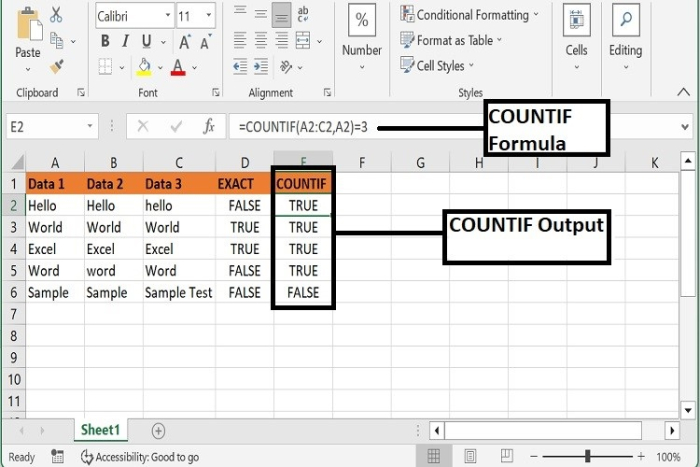## Conclusion

Hence, we have learned two functions to compare the multiple cell values with exact and similar value types. Keep practicing and keep learning excel.

Updated on: 29-Dec-2022

3K+ Views